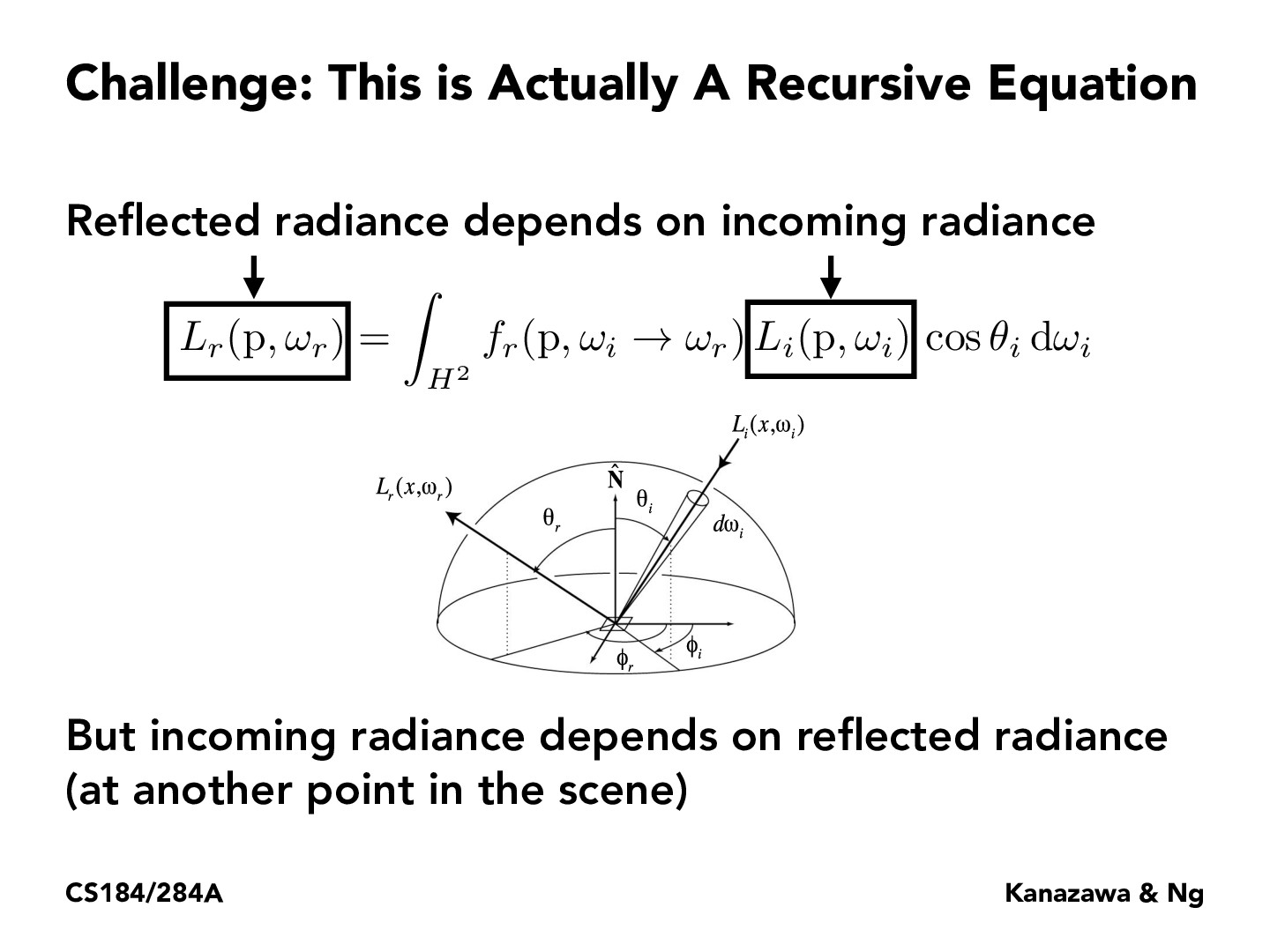Lecture 13: Global Illumination & Path Tracing (29)ronthalanki

Since this equation is recursive, I understand that the computed reflected radiance would increase with each iteration as we plug in the computed reflected radiance back into the equation as the incoming radiance. Would we expect that the equation would converge given the multipliers to the incoming radiance component?aravind00r

The fr co-eff's should stack as we go down the recursive calls, so the radiance values should be getting smaller and smaller as we continue recursing. I think this is what leads to the idea that eventually the incoming radiance will converge to a value.

You must be enrolled in the course to comment